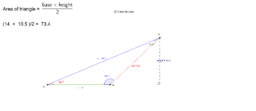•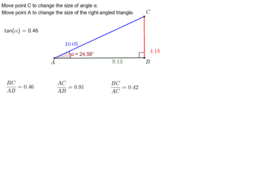### Trigonometric ratio - Tangent

Activity

•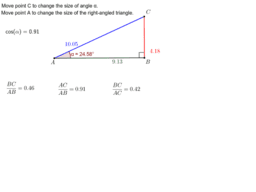### Trigonometric ratio - Cosine

Activity

•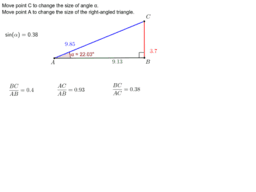### Trigonometric ratio - Sine

Activity

•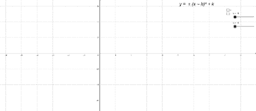Activity

•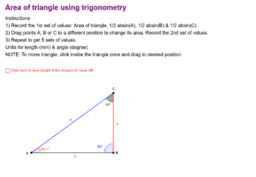### Area of triangle using trigonometry

Activity

•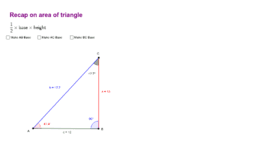### recap on area of triangle 1/2 x base x height

Activity

•